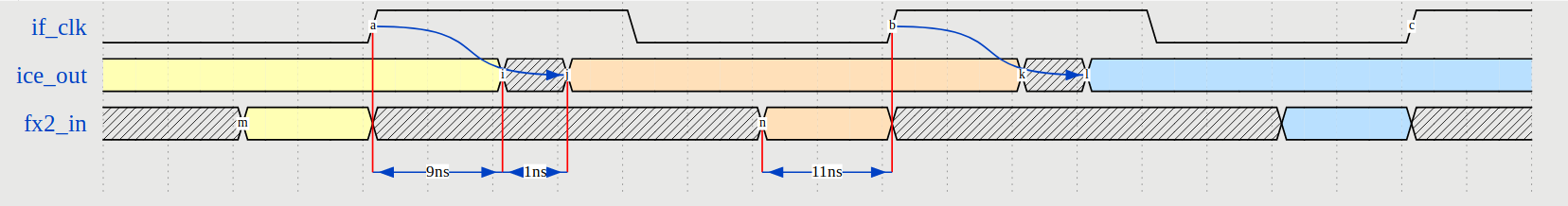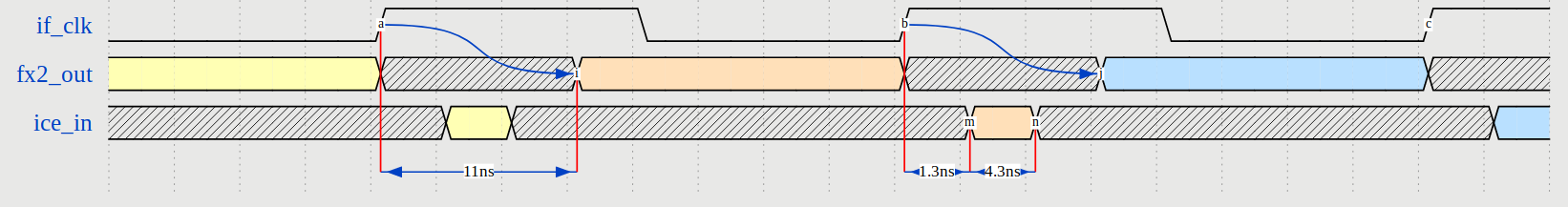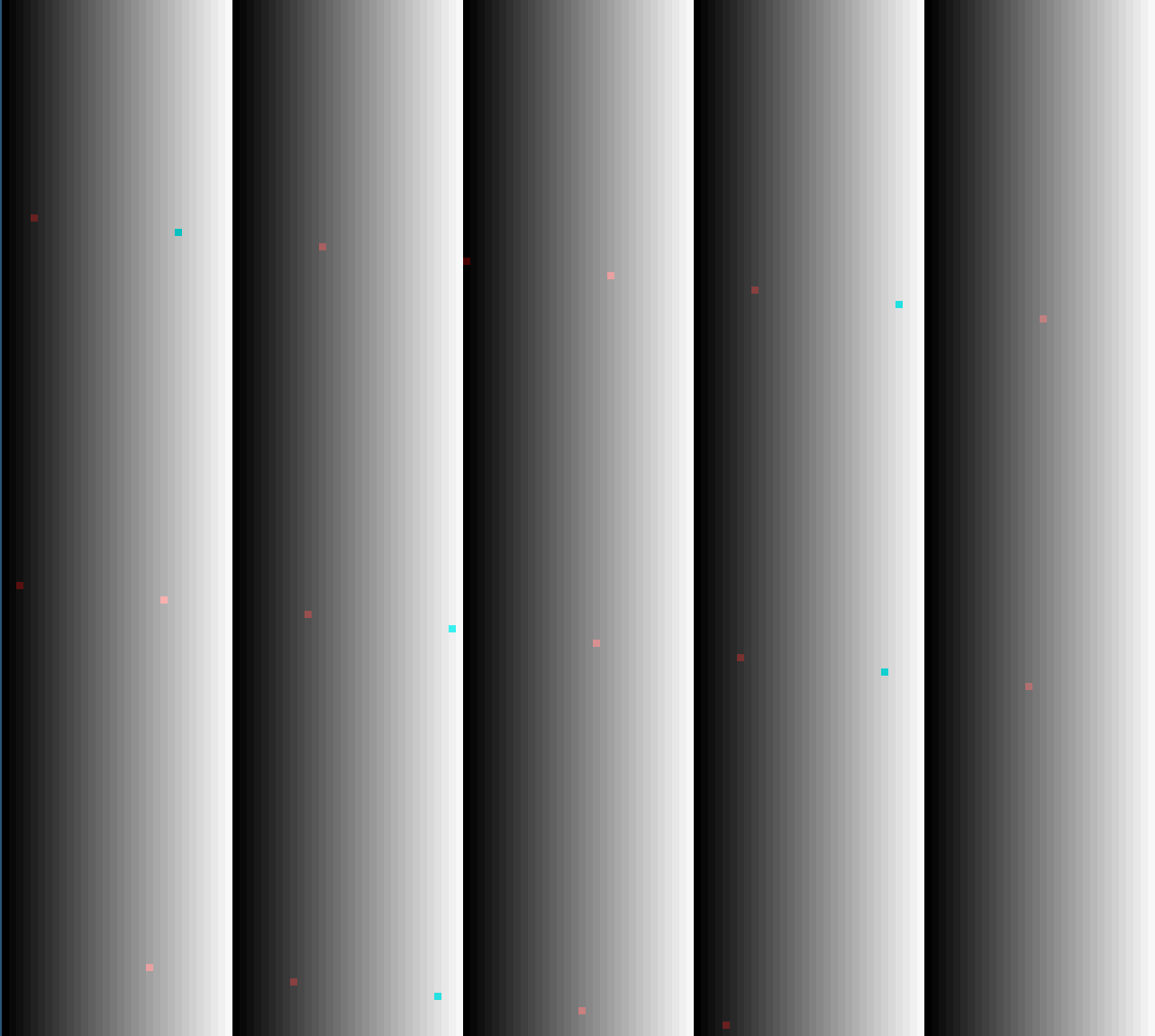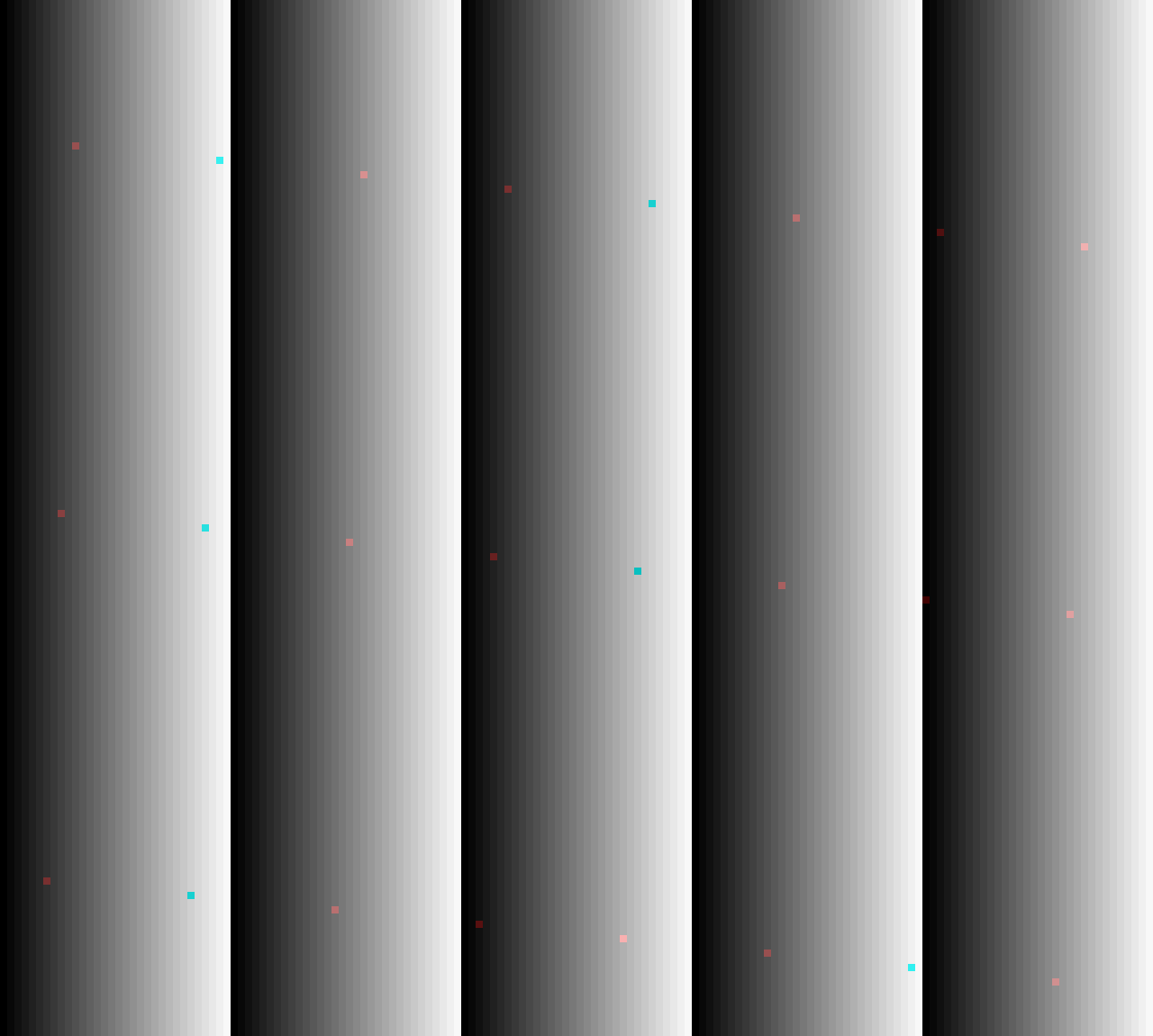/ glasgow Public

# Use SB_GB_IO instead of SB_IO+SB_GB#89

Closed
opened this issue Dec 5, 2018 · 21 comments
Closed

# Use SB_GB_IO instead of SB_IO+SB_GB #89

opened this issue Dec 5, 2018 · 21 comments
Labels
gateware Component: gateware
Milestone

### whitequark commented Dec 5, 2018

 SB_GB_IO has a much lower (and completely predictable!) delay than SB_IO + SB_GB pair we are using now. In fact, using the latter pair has been observed to (rarely) lead to selftest failures due to setup/hold violations on FD pins. Unfortunately, simply switching to SB_GB_IO completely breaks FX2 timing. I have adjusted the strobes to work (without actually trying to think of a proper solution) in the `sb_gb_io` branch, but really, a model of the FX2 bus and tests are necessary to fix this properly. See #44. The text was updated successfully, but these errors were encountered:

### smunaut commented Dec 6, 2018

I've been looking into the timings between the FX2 and ICE interface.

### ICE to FX2

The ICE has a clock-to-out somewhere between 9 and 10 ns. (relative to the `if_clk` at the fpga pad).
The FX2 has requirements for up to 11 ns setup time (depends on which signal) and 0ns hold time.

So this works just fine. The data valid window of the ICE outputs entirely cover the data capture window of the FX2 with plenty of margins. Whatever the ICE outputs will be captured on the next rising clk edge by the FX2.

See diagram below :### FX2 to ICE

The FX2 is a bit under-specified in its clock-to-out. There is only a max value of 11 ns but no minimum value, so we have to assume that the data isn't valid at all from the clock edge up to 11 ns after it.

In the ICE40 it takes a long time for the clock to go from the pad to the actual `SB_IO` register, so the data capture window is well after the clock edge has happened. The setup time is -1.3 ns and hold time is 5.6 ns.

So this means that the ICE40 data capture window is right in the middle of the unstable part of the FX2 output :/ See diagram below.The best way to solve this would be to use the DDR registers in the `SB_IO` to capture the window on the falling edge of `if_clk` and then immediate re-register it in a fabric `DFF` on the rising edge. This way the data would be captured in the valid zone and the fpga logic would see whatever is output by the FX2 on the next rising edge of `if_clk`.

### Details

Attached the timing report from icecube to get details on the IO timings. Also attached the sources for the wavedrom drawings.

### whitequark commented Jan 13, 2019

 Thank you for your analysis again! I'm not entirely sure about one facet here: The best way to solve this would be to use the DDR registers in the `SB_IO` to capture the window on the falling edge of `if_clk` and then immediate re-register it in a fabric `DFF` on the rising edge. Do I understand it correctly that this adds another cycle of latency with regards to the current arrangement with `PIN_INPUT_REGISTERED`?

added a commit that referenced this issue Jan 13, 2019
``` gateware: use SB_GB_IO instead of SB_IO+SB_GB to reduce delay. ```
``` 9f325b8 ```
```Unfortunately, this takes liberties with the FX2 interface; the data
is now captured on falling edge, so instead of one full cycle of
data validity there is only a half cycle. However, the FX2 interface
was certainly broken before, and it seems less broken to me now, so
I am committing this anyway.

Ideally, the FX2 interface would register the input on the next
rising edge. However, this would add two registers in the input path,
and we are already using the INFM1 bit in the FX2. Therefore, adding
this register would require a radical rethinking of the entire FX2
interface.

See #89.```

### whitequark commented Jan 13, 2019

 Looks like my understanding is correct. Therefore, there is a problem with this solution. Namely, what happens when we are filling an IN FIFO and it becomes full? With one register, FX2 has an `INFM1` bit, which causes the FIFO flag (which is also registered like this!) to go low one sample before the FIFO is actually full. But there is no `INFM2` flag! (I'm not sure how `INFM1` actually works--is it effectively the same as using a programmable flag with level set to depth minus two, as opposed to depth minus one? If yes, we could just use a PF then.)

### smunaut commented Jan 13, 2019

 Mmm, that's weird I would have thought that the latency would be the same. If we look at the 'orange' data cycle for the fx2_out in the diagram above. In the previous implementation, I would have assumed that what the ice40 captured during the invalid window was that orange cycle data like I put on the diagram. And so if you capture on the falling edge, you essentially move that capture window half a cycle earlier than currently, and then re-registering it once on the rising edge in the fabric would bring it "in-alignement" with what the `PIN_INPUT_REGISTERED` version was doing previously.

### whitequark commented Feb 9, 2019

 @smunaut I'd like to ask you for a favor. Can you please take another look at the timings of this interface, but this time, assuming externally sourced (in the FPGA) CLKIF, where the CLKIF output is produced using a DDR register with `.D_OUT_0(0)`, `D_OUT_1(1)`. I suspect this might simplify things radically.

### whitequark commented Mar 18, 2019

 @smunaut Bad news: this doesn't help. With the video-rgb-input applet, there is digital snow on the output, which I know for sure is caused by timings mismatch between ICE and FX2. I have a bitstream I made half a year ago that doesn't have this problem but I have no idea how to reproduce it. There's still something else wrong with the arbiter.

### whitequark commented Mar 19, 2019

 Looks like ae5cbc1 is the good commit. Bisecting now.

### whitequark commented Mar 19, 2019

 I've bisected this down to... 630b524. Which is not really of help.

### whitequark commented Apr 2, 2019

 I've made a standalone way to reproduce the glitches. First, apply this patch: ```diff --git a/software/glasgow/applet/video/rgb_input/__init__.py b/software/glasgow/applet/video/rgb_input/__init__.py index aa8ee9d..f472b19 100644 --- a/software/glasgow/applet/video/rgb_input/__init__.py +++ b/software/glasgow/applet/video/rgb_input/__init__.py @@ -3,6 +3,7 @@ import math from migen import * from migen.genlib.cdc import * +from ....gateware.clockgen import * from ... import * @@ -12,12 +13,15 @@ class VideoRGBInputSubtarget(Module): gx = Signal(5) bx = Signal(5) dck = Signal() - self.specials += [ - MultiReg(pads.r_t.i, rx), - MultiReg(pads.g_t.i, gx), - MultiReg(pads.b_t.i, bx), - MultiReg(pads.dck_t.i, dck) - ] + # self.specials += [ + # MultiReg(pads.r_t.i, rx), + # MultiReg(pads.g_t.i, gx), + # MultiReg(pads.b_t.i, bx), + # MultiReg(pads.dck_t.i, dck) + # ] + + self.submodules.clockgen = ClockGen(ClockGen.derive(sys_clk_freq, 1.4e6)) + self.comb += dck.eq(self.clockgen.clk) dck_r = Signal() stb = Signal() @@ -59,7 +63,7 @@ class VideoRGBInputSubtarget(Module): NextState("SKIP-FIRST-PIXEL") ).Elif(row == rows, NextValue(row, 0), - NextState("REPORT-FRAME") + NextState("CAPTURE-ROW") ).Else( NextState("REPORT-FRAME") ) @@ -86,7 +90,7 @@ class VideoRGBInputSubtarget(Module): ("REPORT-3", 10, "CAPTURE-PIXEL") ): self.fsm.act(state, - din.eq((pixel >> offset) & 0x1f), + din.eq(col & 0x1f),#(pixel >> offset) & 0x1f), we.eq(1), NextState(nextstate) )``` Second, run: ``````glasgow run video-rgb-input --port AB --voltage 3.3 --rows 145 --columns 160 --vblank 960e-6 `````` Third, run: ``````git clone https://github.com/whitequark/gameboy-grabber/ cd gameboy-grabber cargo run `````` This is what it should display:This is what it displays:### whitequark commented Apr 2, 2019

 Looks pretty much the same on revB:### whitequark commented Apr 2, 2019

 My question on the Cypress forum: https://community.cypress.com/message/191835

### enjoy-digital commented Apr 2, 2019

 Have you tried eliminating the FIFO datapath from the equation? (If the corruption is on the first valid cycle after interruption, it also means it's the first data outputed by the fifo after being empty, which is also a corner case). I would personally do a test by keeping the exact same control flow and just replace the FIFO data that is going to the FX2 with a counter and see if you also have the corruption. (but maybe you already verified that).

### whitequark commented Apr 2, 2019

 @enjoy-digital You are right! There's no corruption if I replace the FIFO with a counter. But... the FIFO is just migen's SyncFIFOBuffered...

### enjoy-digital commented Apr 2, 2019 • edited

 Then it seems there is a mismatch between the control/data path for this corner case on the FIFO. I'm using the same FIFO on several designs in situations that are very similar (mostly on Xilinx devices). Maybe something is not handled correctly in the toolchain. At least it shows the issue is probably not on the FX2 side.

### whitequark commented Apr 2, 2019

 Yeah...

### daveshah1 commented Apr 2, 2019

 Do you have YosysHQ/yosys#896 in your Yosys tree?

### whitequark commented Apr 2, 2019

 @daveshah1 If I use Yosys master instead of whichever old commit I've had, it segfaults.

### whitequark commented Apr 2, 2019

 @gregdavill Repro: bug.zip

### daveshah1 commented Apr 2, 2019

 Hmm, can't reproduce and Valgrind shows nothing either over here. But it doesn't hit the #896 case either, so that's not related anyway.

### whitequark commented Apr 2, 2019

 @daveshah1 Valgrind log of the crash: ``````3.10.6. Executing OPT_RMDFF pass (remove dff with constant values). ==7696== Conditional jump or move depends on uninitialised value(s) ==7696== at 0x66DE72: std::_Rb_tree, std::less, std::allocator >::_M_erase(std::_Rb_tree_node*) (stl_tree.h:1870) ==7696== by 0x66DFB5: std::_Rb_tree, std::less, std::allocator >::~_Rb_tree() (stl_tree.h:965) ==7696== by 0x877D60: ~set (stl_set.h:281) ==7696== by 0x877D60: ~pair (stl_pair.h:193) ==7696== by 0x877D60: ~entry_t (hashlib.h:202) ==7696== by 0x877D60: _Destroy >::bitDef_t, std::set, std::allocator >, Yosys::hashlib::hash_ops >::bitDef_t> >::entry_t> (stl_construct.h:98) ==7696== by 0x877D60: __destroy >::bitDef_t, std::set, std::allocator >, Yosys::hashlib::hash_ops >::bitDef_t> >::entry_t *> (stl_construct.h:108) ==7696== by 0x877D60: _Destroy >::bitDef_t, std::set, std::allocator >, Yosys::hashlib::hash_ops >::bitDef_t> >::entry_t *> (stl_construct.h:136) ==7696== by 0x877D60: _Destroy >::bitDef_t, std::set, std::allocator >, Yosys::hashlib::hash_ops >::bitDef_t> >::entry_t *, Yosys::hashlib::dict >::bitDef_t, std::set, std::allocator >, Yosys::hashlib::hash_ops >::bitDef_t> >::entry_t> (stl_construct.h:206) ==7696== by 0x877D60: _M_erase_at_end (stl_vector.h:1658) ==7696== by 0x877D60: clear (stl_vector.h:1386) ==7696== by 0x877D60: clear (hashlib.h:557) ==7696== by 0x877D60: Yosys::SigSet >::clear() (sigtools.h:151) ==7696== by 0x872BA3: (anonymous namespace)::OptRmdffPass::execute(std::vector, std::allocator >, std::allocator, std::allocator > > >, Yosys::RTLIL::Design*) (opt_rmdff.cc:542) ==7696== by 0x52E658: Yosys::Pass::call(Yosys::RTLIL::Design*, std::vector, std::allocator >, std::allocator, std::allocator > > >) (register.cc:234) ==7696== by 0x52E2AB: Yosys::Pass::call(Yosys::RTLIL::Design*, std::__cxx11::basic_string, std::allocator >) (register.cc:214) ==7696== by 0x85EA69: (anonymous namespace)::OptPass::execute(std::vector, std::allocator >, std::allocator, std::allocator > > >, Yosys::RTLIL::Design*) (opt.cc:150) ==7696== by 0x52E658: Yosys::Pass::call(Yosys::RTLIL::Design*, std::vector, std::allocator >, std::allocator, std::allocator > > >) (register.cc:234) ==7696== by 0x52E2AB: Yosys::Pass::call(Yosys::RTLIL::Design*, std::__cxx11::basic_string, std::allocator >) (register.cc:214) ==7696== by 0x52EECF: Yosys::ScriptPass::run(std::__cxx11::basic_string, std::allocator >, std::__cxx11::basic_string, std::allocator >) (register.cc:322) ==7696== by 0x9E296C: (anonymous namespace)::SynthIce40Pass::script() (synth_ice40.cc:240) ==7696== by 0x9E21E5: (anonymous namespace)::SynthIce40Pass::execute(std::vector, std::allocator >, std::allocator, std::allocator > > >, Yosys::RTLIL::Design*) (synth_ice40.cc:214) ==7696== ==7696== Use of uninitialised value of size 8 ==7696== at 0x66DE74: _S_right (stl_tree.h:788) ==7696== by 0x66DE74: std::_Rb_tree, std::less, std::allocator >::_M_erase(std::_Rb_tree_node*) (stl_tree.h:1872) ==7696== by 0x66DFB5: std::_Rb_tree, std::less, std::allocator >::~_Rb_tree() (stl_tree.h:965) ==7696== by 0x877D60: ~set (stl_set.h:281) ==7696== by 0x877D60: ~pair (stl_pair.h:193) ==7696== by 0x877D60: ~entry_t (hashlib.h:202) ==7696== by 0x877D60: _Destroy >::bitDef_t, std::set, std::allocator >, Yosys::hashlib::hash_ops >::bitDef_t> >::entry_t> (stl_construct.h:98) ==7696== by 0x877D60: __destroy >::bitDef_t, std::set, std::allocator >, Yosys::hashlib::hash_ops >::bitDef_t> >::entry_t *> (stl_construct.h:108) ==7696== by 0x877D60: _Destroy >::bitDef_t, std::set, std::allocator >, Yosys::hashlib::hash_ops >::bitDef_t> >::entry_t *> (stl_construct.h:136) ==7696== by 0x877D60: _Destroy >::bitDef_t, std::set, std::allocator >, Yosys::hashlib::hash_ops >::bitDef_t> >::entry_t *, Yosys::hashlib::dict >::bitDef_t, std::set, std::allocator >, Yosys::hashlib::hash_ops >::bitDef_t> >::entry_t> (stl_construct.h:206) ==7696== by 0x877D60: _M_erase_at_end (stl_vector.h:1658) ==7696== by 0x877D60: clear (stl_vector.h:1386) ==7696== by 0x877D60: clear (hashlib.h:557) ==7696== by 0x877D60: Yosys::SigSet >::clear() (sigtools.h:151) ==7696== by 0x872BA3: (anonymous namespace)::OptRmdffPass::execute(std::vector, std::allocator >, std::allocator, std::allocator > > >, Yosys::RTLIL::Design*) (opt_rmdff.cc:542) ==7696== by 0x52E658: Yosys::Pass::call(Yosys::RTLIL::Design*, std::vector, std::allocator >, std::allocator, std::allocator > > >) (register.cc:234) ==7696== by 0x52E2AB: Yosys::Pass::call(Yosys::RTLIL::Design*, std::__cxx11::basic_string, std::allocator >) (register.cc:214) ==7696== by 0x85EA69: (anonymous namespace)::OptPass::execute(std::vector, std::allocator >, std::allocator, std::allocator > > >, Yosys::RTLIL::Design*) (opt.cc:150) ==7696== by 0x52E658: Yosys::Pass::call(Yosys::RTLIL::Design*, std::vector, std::allocator >, std::allocator, std::allocator > > >) (register.cc:234) ==7696== by 0x52E2AB: Yosys::Pass::call(Yosys::RTLIL::Design*, std::__cxx11::basic_string, std::allocator >) (register.cc:214) ==7696== by 0x52EECF: Yosys::ScriptPass::run(std::__cxx11::basic_string, std::allocator >, std::__cxx11::basic_string, std::allocator >) (register.cc:322) ==7696== by 0x9E296C: (anonymous namespace)::SynthIce40Pass::script() (synth_ice40.cc:240) ==7696== by 0x9E21E5: (anonymous namespace)::SynthIce40Pass::execute(std::vector, std::allocator >, std::allocator, std::allocator > > >, Yosys::RTLIL::Design*) (synth_ice40.cc:214) ==7696== ==7696== Invalid read of size 8 ==7696== at 0x66DE74: _S_right (stl_tree.h:788) ==7696== by 0x66DE74: std::_Rb_tree, std::less, std::allocator >::_M_erase(std::_Rb_tree_node*) (stl_tree.h:1872) ==7696== by 0x66DFB5: std::_Rb_tree, std::less, std::allocator >::~_Rb_tree() (stl_tree.h:965) ==7696== by 0x877D60: ~set (stl_set.h:281) ==7696== by 0x877D60: ~pair (stl_pair.h:193) ==7696== by 0x877D60: ~entry_t (hashlib.h:202) ==7696== by 0x877D60: _Destroy >::bitDef_t, std::set, std::allocator >, Yosys::hashlib::hash_ops >::bitDef_t> >::entry_t> (stl_construct.h:98) ==7696== by 0x877D60: __destroy >::bitDef_t, std::set, std::allocator >, Yosys::hashlib::hash_ops >::bitDef_t> >::entry_t *> (stl_construct.h:108) ==7696== by 0x877D60: _Destroy >::bitDef_t, std::set, std::allocator >, Yosys::hashlib::hash_ops >::bitDef_t> >::entry_t *> (stl_construct.h:136) ==7696== by 0x877D60: _Destroy >::bitDef_t, std::set, std::allocator >, Yosys::hashlib::hash_ops >::bitDef_t> >::entry_t *, Yosys::hashlib::dict >::bitDef_t, std::set, std::allocator >, Yosys::hashlib::hash_ops >::bitDef_t> >::entry_t> (stl_construct.h:206) ==7696== by 0x877D60: _M_erase_at_end (stl_vector.h:1658) ==7696== by 0x877D60: clear (stl_vector.h:1386) ==7696== by 0x877D60: clear (hashlib.h:557) ==7696== by 0x877D60: Yosys::SigSet >::clear() (sigtools.h:151) ==7696== by 0x872BA3: (anonymous namespace)::OptRmdffPass::execute(std::vector, std::allocator >, std::allocator, std::allocator > > >, Yosys::RTLIL::Design*) (opt_rmdff.cc:542) ==7696== by 0x52E658: Yosys::Pass::call(Yosys::RTLIL::Design*, std::vector, std::allocator >, std::allocator, std::allocator > > >) (register.cc:234) ==7696== by 0x52E2AB: Yosys::Pass::call(Yosys::RTLIL::Design*, std::__cxx11::basic_string, std::allocator >) (register.cc:214) ==7696== by 0x85EA69: (anonymous namespace)::OptPass::execute(std::vector, std::allocator >, std::allocator, std::allocator > > >, Yosys::RTLIL::Design*) (opt.cc:150) ==7696== by 0x52E658: Yosys::Pass::call(Yosys::RTLIL::Design*, std::vector, std::allocator >, std::allocator, std::allocator > > >) (register.cc:234) ==7696== by 0x52E2AB: Yosys::Pass::call(Yosys::RTLIL::Design*, std::__cxx11::basic_string, std::allocator >) (register.cc:214) ==7696== by 0x52EECF: Yosys::ScriptPass::run(std::__cxx11::basic_string, std::allocator >, std::__cxx11::basic_string, std::allocator >) (register.cc:322) ==7696== by 0x9E296C: (anonymous namespace)::SynthIce40Pass::script() (synth_ice40.cc:240) ==7696== by 0x9E21E5: (anonymous namespace)::SynthIce40Pass::execute(std::vector, std::allocator >, std::allocator, std::allocator > > >, Yosys::RTLIL::Design*) (synth_ice40.cc:214) ==7696== Address 0x100000017 is not stack'd, malloc'd or (recently) free'd ==7696== ==7696== ==7696== Process terminating with default action of signal 11 (SIGSEGV) ==7696== Access not within mapped region at address 0x100000017 ==7696== at 0x66DE74: _S_right (stl_tree.h:788) ==7696== by 0x66DE74: std::_Rb_tree, std::less, std::allocator >::_M_erase(std::_Rb_tree_node*) (stl_tree.h:1872) ==7696== by 0x66DFB5: std::_Rb_tree, std::less, std::allocator >::~_Rb_tree() (stl_tree.h:965) ==7696== by 0x877D60: ~set (stl_set.h:281) ==7696== by 0x877D60: ~pair (stl_pair.h:193) ==7696== by 0x877D60: ~entry_t (hashlib.h:202) ==7696== by 0x877D60: _Destroy >::bitDef_t, std::set, std::allocator >, Yosys::hashlib::hash_ops >::bitDef_t> >::entry_t> (stl_construct.h:98) ==7696== by 0x877D60: __destroy >::bitDef_t, std::set, std::allocator >, Yosys::hashlib::hash_ops >::bitDef_t> >::entry_t *> (stl_construct.h:108) ==7696== by 0x877D60: _Destroy >::bitDef_t, std::set, std::allocator >, Yosys::hashlib::hash_ops >::bitDef_t> >::entry_t *> (stl_construct.h:136) ==7696== by 0x877D60: _Destroy >::bitDef_t, std::set, std::allocator >, Yosys::hashlib::hash_ops >::bitDef_t> >::entry_t *, Yosys::hashlib::dict >::bitDef_t, std::set, std::allocator >, Yosys::hashlib::hash_ops >::bitDef_t> >::entry_t> (stl_construct.h:206) ==7696== by 0x877D60: _M_erase_at_end (stl_vector.h:1658) ==7696== by 0x877D60: clear (stl_vector.h:1386) ==7696== by 0x877D60: clear (hashlib.h:557) ==7696== by 0x877D60: Yosys::SigSet >::clear() (sigtools.h:151) ==7696== by 0x872BA3: (anonymous namespace)::OptRmdffPass::execute(std::vector, std::allocator >, std::allocator, std::allocator > > >, Yosys::RTLIL::Design*) (opt_rmdff.cc:542) ==7696== by 0x52E658: Yosys::Pass::call(Yosys::RTLIL::Design*, std::vector, std::allocator >, std::allocator, std::allocator > > >) (register.cc:234) ==7696== by 0x52E2AB: Yosys::Pass::call(Yosys::RTLIL::Design*, std::__cxx11::basic_string, std::allocator >) (register.cc:214) ==7696== by 0x85EA69: (anonymous namespace)::OptPass::execute(std::vector, std::allocator >, std::allocator, std::allocator > > >, Yosys::RTLIL::Design*) (opt.cc:150) ==7696== by 0x52E658: Yosys::Pass::call(Yosys::RTLIL::Design*, std::vector, std::allocator >, std::allocator, std::allocator > > >) (register.cc:234) ==7696== by 0x52E2AB: Yosys::Pass::call(Yosys::RTLIL::Design*, std::__cxx11::basic_string, std::allocator >) (register.cc:214) ==7696== by 0x52EECF: Yosys::ScriptPass::run(std::__cxx11::basic_string, std::allocator >, std::__cxx11::basic_string, std::allocator >) (register.cc:322) ==7696== by 0x9E296C: (anonymous namespace)::SynthIce40Pass::script() (synth_ice40.cc:240) ==7696== by 0x9E21E5: (anonymous namespace)::SynthIce40Pass::execute(std::vector, std::allocator >, std::allocator, std::allocator > > >, Yosys::RTLIL::Design*) (synth_ice40.cc:214) ==7696== If you believe this happened as a result of a stack ==7696== overflow in your program's main thread (unlikely but ==7696== possible), you can try to increase the size of the ==7696== main thread stack using the --main-stacksize= flag. ==7696== The main thread stack size used in this run was 134217728. ==7696== ==7696== HEAP SUMMARY: ==7696== in use at exit: 4,250,144 bytes in 36,777 blocks ==7696== total heap usage: 1,017,132 allocs, 980,355 frees, 66,737,353 bytes allocated ==7696== ==7696== LEAK SUMMARY: ==7696== definitely lost: 3,328 bytes in 13 blocks ==7696== indirectly lost: 1,144 bytes in 26 blocks ==7696== possibly lost: 0 bytes in 0 blocks ==7696== still reachable: 4,245,672 bytes in 36,738 blocks ==7696== suppressed: 0 bytes in 0 blocks ==7696== Rerun with --leak-check=full to see details of leaked memory ==7696== ==7696== For counts of detected and suppressed errors, rerun with: -v ==7696== Use --track-origins=yes to see where uninitialised values come from ==7696== ERROR SUMMARY: 7 errors from 3 contexts (suppressed: 0 from 0) Segmentation fault ``````

### whitequark commented Apr 2, 2019

 @daveshah1 Nevermind, I rebuilt Yosys and the crash came away.